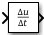# Derivative

•## 说明

Derivative 模块可近似计算输入信号 u 相对于仿真时间 t 的导数。您将获得以下算式的近似值：

`$\frac{du}{dt},$`

`$y\left(t\right)=\frac{\Delta u}{\Delta t}=\frac{u\left(t\right)-u\left({T}_{previous}\right)}{t-{T}_{previous}}|t>{T}_{previous},$`

Derivative 模块的输出可能对整个模型的动态比较敏感。输出信号的准确度取决于仿真中采用的时间步长的大小。步长越小，此模块的输出曲线就越平滑和准确。但是，与具有连续状态的模块不同，当此模块的输入快速变化时，求解器不会采用较小的步长。根据驱动信号和模型的动态，此模块的输出信号可能包含意外波动。这些波动主要是由驱动信号输出和求解器步长导致的。

`$y\left(k\right)=\frac{1}{\Delta t}\left(u\left(k\right)-u\left(k-1\right)\right)$`

`$\frac{Y\left(z\right)}{u\left(z\right)}=\frac{1-{z}^{-1}}{\Delta t}=\frac{z-1}{\Delta t\cdot z}.$`

Discrete Derivative 模块可对此行为进行建模。使用此模块而不是 Derivative 模块可以近似计算离散信号的离散时间导数。

## 端口

### 输出

`$y\left(t\right)=\frac{\Delta u}{\Delta t}=\frac{u\left(t\right)-u\left({T}_{previous}\right)}{t-{T}_{previous}}|t>{T}_{previous},$`

## 参数

Derivative 模块的准确线性化很难，因为该模块的动态方程为 $y=\stackrel{˙}{u}$，无法将其表示为状态空间方程组。但是，您可以接近线性化，方法是为 Derivative 模块添加极点以创建传递函数 $s/\left(c\ast s+1\right).$ 添加极点会在对信号进行微分之前对其滤波，这可以去掉噪声的影响。

#### 提示

• 最佳做法是将 c 的值更改为 $\frac{1}{{f}_{b}}$，其中 ${f}_{b}$ 是滤波器的折转频率。

• 参数必须是有限正值。

#### 编程用法

 模块参数：`CoefficientInTFapproximation` 类型：字符向量、字符串 值：`'inf'` 默认值： `'inf'`

## 模块特性

 数据类型 `double` 直接馈通 `否` 多维信号 `否` 可变大小信号 `否` 过零检测 `否`